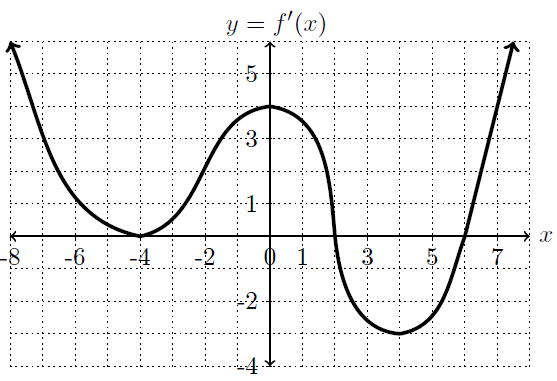## Shape of a Graph            Practice Curve Sketching

### 7. Use the graph of $$y=f'(x)$$ below to answer the following questions about the function $$f$$.   solution